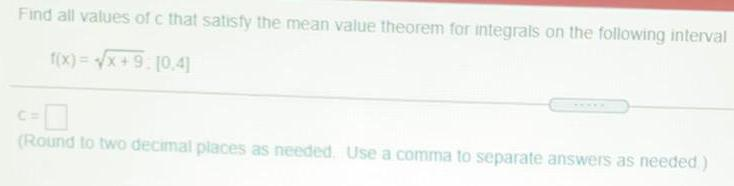Question:

# Find all values of c that satisfy the mean value theorem for integrals on the following interval f(x)=√x+9 [0.4] (Round to two decimal places as needed. Use a comma to separate answers as needed)

Last updated: 7/28/2022Find all values of c that satisfy the mean value theorem for integrals on the following interval f(x)=√x+9 . [0.4]